# Mathematics 1010 online

## Fractions

A fraction is an expression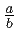where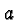and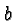are integers, and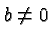. On this page we review some basic facts about fractions. We will also see why these facts are true. The way we'll approach the issues will be typical for approaching the kind of mathematics you will learn in great depth in this class.

You are familiar with these facts:

1. The numberon top of the fraction bar is the numerator, and the numberat the bottom is the denominator of the fraction.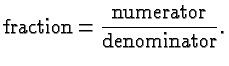2. An integer can be considered a fraction with a denominator equal to 1.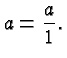3. Multiplying numerator and denominator of a fraction with the same non-zero factor does not change the fraction. (In elementary school the term equivalent fractions is used, but a more mature view is that equivalent fractions denote the same real number, and hence need not be distinguished other than for clarity and simplicity.)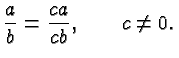4. Fractions with the same denominator are added (or subtracted) by adding (or subtracting) the numerators (and keeping the denominator the same).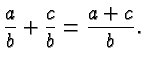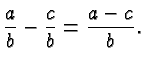5. Fractions with different denominators are first turned into fractions with the same denominators, by multiplying numerators and denominators with suitable factors, and then added using the rule above.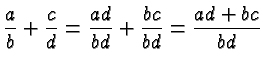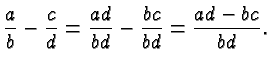6. Fractions are multiplied by multiplying numerators and multiplying denominators.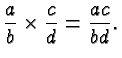7. The reciprocal of a fraction is obtained by switching numerators and denominators.

8. Dividing a fraction by another fraction is equivalent to multiplying with the reciprocal of the second fraction.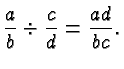Note how we explain the addition of fractions with distinct denominators in terms of the addition of fractions with the same denominator, and the division of fractions in terms of the multiplication of fractions.

These provide instances of a principle whose statement is deceptively simple, but whose applications and implications are far reaching:

Reduce your problem to one you have solved before.

### What is a fraction?

A fraction such as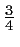is simply a way to denote the result of dividing 3 by 4. In general, a fractiondenotes the result of dividingby. (This is true even ifandaren't integers, an illustration of the principle that we make definitions in simple contexts and then generalize them so that all relevant rules remain true. However, ifandaren't integer then the expressionis called a ratio or quotient.)

Division is defined as the solution of a multiplication problem. Thus the fraction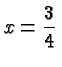is the solution of the equation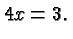More generally, the fraction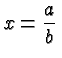is the solution of the equation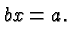.

It's important to understand this basic definition, since all of the above rules can be derived from it.

We do, however, require another principle

Doing the same on both sides of an equation creates another valid equation.:

### Multiplying Numerator and Denominator with the Same Factor

The solution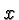of the equation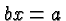does not change if we multiply on the left and right with the same factor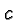to obtain the equivalent equation. We have as an immediate consequence the above identity:Of course, this equation can be read from right to left just as easily as from left to right, which provides the basis for canceling common factors or reducing to lowest terms.

### Adding Fractions with Like Denominators.

Let's suppose we are given two fractions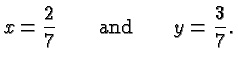As we discussed, these fractions are defined by the equations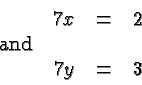We now add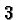on both sides of the first equation. This will give another valid equation. However, on the left we will not use the number, but instead the expression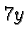. We can do this because the second equation asserts thatequals. Henceforth we will simply say that we add the second equation to the first, but the underlying reasoning is that we add the same thing on both sides of the first equation, we just give it different names on the two sides of the equation. This operation gives the new equation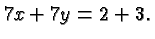It can be rewritten as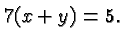By the definition of fractions this amounts to the statement that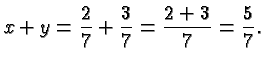It is clear how this argument applies to general fractions with the same denominator, and to the subtraction (as opposed to the addition) of fractions.

### Adding Fractions with Unlike Denominators

When adding two fractions like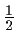and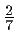we first convert them to fractions with the same denominator by applying rule number 3 above. Thus we have to find a common denominator. As a practical matter, the smaller the denominator the easier it is to manipulate the numbers, and therefore we like to use the least common denominator. However, the product of the two denominators always works, and it is often, like in this case, also the least common denominator. So in this example we use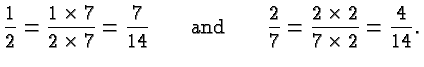Thus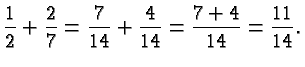### Multiplying Fractions

Suppose we are given two fractions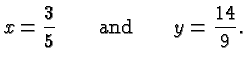This meansand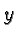satisfy the equations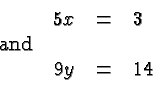Proceeding as above, and multiplying on both sides of the first equation with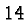, but calling it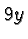on the left, we obtain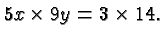This can be rewritten as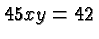which is another way of saying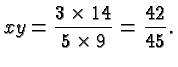Of course we can simplify that fraction by dividing numerator and denominator by 3: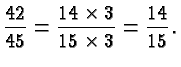### Dividing fractions

Suppose we want to divide the same fractions as above. So we ask what is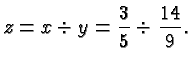Division is the inverse process of multiplication, so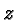satisfies the equation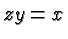or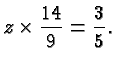The answer can be obtained by multiplying on both sides of this equation with the reciprocal of, i.e., with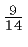.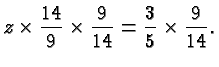This becomes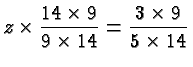which simplifies to### Greatest Common Factors and Least Common Multiples

A multiple of a natural number is the product of that number with another natural number. For example, 12, 15, and 333333 are all multiples of 3. A factor of a natural number n is another natural number that divides n without remainder. For example, (all) the factors of 12 are 1, 2, 3, 4, 6, 12. A common factor of two natural numbers m and n is a number that is a factor of m and also of n. For example, 8 is a common factor of 32 and 48. Other common factors are 1, 2, 4, 8, 16. The greatest common factor is just what the name suggests, so the greatest common factor of 32 and 48 is 16. Similarly, a common multiple of two numbers is a multiple of both, and the least common multiple is the smallest common multiple. For example, 24, 120, 180 are all common multiples of 4 and 6, and 12 is the least common multiple.

The relevance of all this is that a common denominator of two fractions is a common multiple of the two denominators, and the least common denominator is the least common multiple of the two denominators.

The least common multiple LCM and the greatest common factor GCF of two numbers m and n are related by the fact

LCM = m*n/GCF
For example, the GCF of 4 and 6 is 2, their least common multiple is 12 and indeed
 12 = 4*6/2

### Mixed Numbers

Mixed Numbers are fractions written as a natural number plus a fraction where the denominator is greater than the numerator. For example,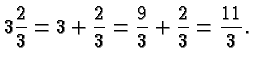Mixed numbers are popular because the integer part gives an indication of their size, but otherwise they have little to recommend them. They form an exception (the only exception) to the rule that a missing operator means multiplication, and they make the arithmetic operations harder to carry out. We will not use them in this class and I recommend you ignore their existence.

### Divisibility Rules

When working with fractions it is convenient to eliminate common factors in numerator and denominator, to keep numerators and denominators as small as possible.

For large numerators and denominators the most practical way of finding common factors is the Euclidean Algorithm described elsewhere, but for many small factors there are simple rules available. They are listed in the following Table. It's a good exercise to think about why these rules hold. If you can't figured it out drop me a note!

 Factor Rule Examples 2 last digit is 0, 2, 4, 6, or 8 2, or 127174 3 sum of digits is divisible by 3 111 (s.o.d. = 1+1+1 = 3.) 111= 3*37, or 212,319,231 (s.o.d. = 24), 212,319,231 = 3*70,773,077. 4 the last two digits form a number that is divisible by 4. 1,232, or 12,135,432,196 5 last digit is 0, or 5 58,213,475 6 Apply tests for 2 and 3 228, or 5,832 7 there is no good test, divide by 7 2,443 8 the last three digits form a number that is divisible by 8. 25,432, or 2,942,600 9 sum of digits is divisible by 9 111 (s.o.d. = 3+3+3 =9.) 333= 9*37. 242,319,231 (s.o.d. = 27), or 242,319,231 = 9*26,924,359. 10 last digit is 0 20, or 123,456,780

There is also a well known rule for divisibility by 11. You form one sum by adding the first, third, fifth, etc. digit, and another by adding the second, fourth, sixth, etc. The number is divisible by 11 is the difference of the sums is. For example, suppose we want to check

m = 5,123,456,789.

The sum of the digits in the odd numbered positions is 9+7+5+3+1 = 25. The sum of the digits in the even numbered positions is 8+6+4+2+5 = 25. The difference is 25-25 = 0. 0 is divisible by 11, and so is m. Indeed,

5,123,456,789 = 11*465,768,799.

Check it out!